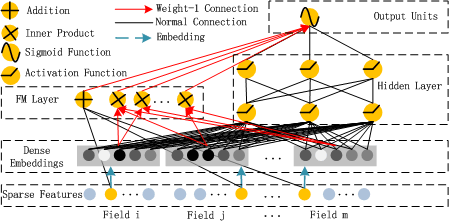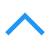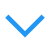# 因子分解机 FM

## 特征组合

• 特征爆炸
• 大量重要的特征组合都隐藏在数据中，无法被专家识别和设计

1. 广度模型包括FM/FFM等大规模低秩(Low-Rank)模型，FM/FFM通过对特征的低秩展开，为每个特征构建隐式向量，并通过隐式向量的点乘结果来建模两个特征的组合关系实现对二阶特征组合的自动学习。作为另外一种模型，Poly-2模型则直接对2阶特征组合建模来学习它们的权重。FM/FFM相比于Poly-2模型，优势为以下两点。第一，FM/FFM模型所需要的参数个数远少于Poly-2模型：FM/FFM模型为每个特征构建一个隐式向量，所需要的参数个数为 $O(km)$，其中k为隐式向量维度，m为特征个数；Poly-2模型为每个2阶特征组合设定一个参数来表示这个2阶特征组合的权重，所需要的参数个数为 $O(m^2)$。第二，相比于Poly-2模型，FM/FFM模型能更有效地学习参数：当一个2阶特征组合没有出现在训练集时，Poly-2模型则无法学习该特征组合的权重；但是FM/FFM却依然可以学习，因为该特征组合的权重是由这2个特征的隐式向量点乘得到的，而这2个特征的隐式向量可以由别的特征组合学习得到。总体来说，FM/FFM是一种非常有效地对二阶特征组合进行自动学习的模型。
2. 深度学习是通过神经网络结构和非线性激活函数，自动学习特征之间复杂的组合关系。目前在APP推荐领域中比较流行的深度模型有FNN/PNN/Wide & Deep。FNN模型是用FM模型来对Embedding层进行初始化的全连接神经网络。PNN模型则是在Embedding层和全连接层之间引入了内积/外积层，来学习特征之间的交互关系。Wide & Deep模型由谷歌提出，将LR和DNN联合训练，在Google Play取得了线上效果的提升。

## FM 因子分解

FM算法可以在线性时间内完成模型训练, 是一个非常高效的模型。FM最大特点和优势：FM模型对稀疏数据有更好的学习能力，通过交互项可以学习特征之间的关联关系，并且保证了学习效率和预估能力。
One-Hot编码的特点: 大部分样本的特征比较稀疏; 特征空间大。

$y(x)=w_0+\sum_{i=1}^nw_ix_i+\sum_{i=1}^n\sum_{j=i+1}^nw_{ij}x_ix_j$

$y(x)=w_0+\sum _{i=1}^nw_ix_i+\sum_{i=1}^n\sum_{j=i+1}^n⟨vi,vj⟩x_ix_j \\ ⟨v_i,v_j⟩=\sum_{f=1}^kv_{i,f}·v_{j,f}$

$\sum_{i=1}^n\sum_{j=i+1}^n⟨v_i,v_j⟩x_i,x_j=\frac{1}{2}\sum_{f=1}^k[(\sum_{i=1}^nv_{i,f}x_i)^2-\sum_{i=1}^nv_{i,f}^2x_i^2]$

\begin{align} &\sum_{i=1}^n\sum_{j=i+1}^n⟨v_i,v_j⟩x_ix_j \\ =&\frac{1}{2}\sum_{i=1}^n\sum_{j=1}^n⟨v_i,v_j⟩x_ix_j-\frac{1}{2}\sum_{i=1}^n⟨v_i,v_i⟩x_ix_i \\ =&\frac{1}{2}(\sum_{i=1}^n\sum_{j=1}^n\sum_{f=1}^kv_{i,f}v_{j,f}x_ix_j-\sum_{i=1}^n\sum_{f=1}^kv_{i,f}v_{i,f}x_ix_i) \\ =&\frac{1}{2}\sum_{f=1}^k[(\sum_{i=1}^nv_{i,f}x_i)·(\sum_{j=1}^nv_{j,f}x_j)-\sum_{i=1}^nv_{i,f}^2x_i^2] \\ =&\frac{1}{2}\sum_{f=1}^k[(\sum_{i=1}^nv_{i,f}x_i)^2- \sum_{i=1}^nv_{i,f}^2x_i^2] \end{align}

FM模型的核心作用可以概括为以下三个：

1. FM降低了交叉项参数学习不充分的影响：one-hot编码后的样本数据非常稀疏，组合特征更是如此。为了解决交叉项参数学习不充分、导致模型有偏或不稳定的问题。作者借鉴矩阵分解的思路：每一维特征用k维的隐向量表示，交叉项的参数$w_{ij}$用对应特征隐向量的内积表示，即$⟨v_i,v_j⟩$。这样参数学习由之前学习交叉项参数$w_{ij}$的过程，转变为学习n个单特征对应k维隐向量的过程。
2. FM提升了模型预估能力。可以用于预估训练集中没有出现过的特征组合项.

• FM是一种比较灵活的模型，通过合适的特征变换方式，FM可以模拟二阶多项式核的SVM模型、MF模型、SVD++模型等；
• 相比SVM的二阶多项式核而言，FM在样本稀疏的情况下是有优势的；而且，FM的训练/预测复杂度是线性的，而二项多项式核SVM需要计算核矩阵，核矩阵复杂度就是N平方。

## FFM（场感知分解机,Field-aware Factorization Machine）

FM的缺点: 由于需要两两组合特征, 这样任意两个交叉特征之间都有了直接或者间接的关联, 因此任意两组特征交叉组合的隐向量都是相关的, 这实际上限制了模型的复杂度. 但是如果使得任意一对特征组合都是完全独立的, 这与通过核函数计算特征交叉类似, 有着极高的复杂性和自由度, 模型计算十分复杂. FFM正好介于这两者之间.
FFM引入特征组(field)的概念来优化此问题. FFM把相同性质的特征归于同一个field, 按照级别分别计算当前特征与其它field的特征组合时的特征向量, 这样特征组合的数量将大大减少.

$y(x)=w_0+\sum_{i=1}^nw_ix_i+\sum_{i=1}^n\sum_{j=i+1}^n⟨v_{i,fj},v_{j,f_i}⟩x_ix_j \\$

$ϕ(w,x)=\sum_{j1,j2∈C_2}⟨w_{j_1,f_2},w_{j_2,f_1}⟩x_{j_1}x_{j_2}$

$\underset{w}{\min}\sum_{i=1}^n\log(1+\exp{−y_iϕ(w,x_i)})+\frac{λ}{2}‖w‖^2$

## FwFM

FFM相当于在FM的基础上考虑了不同域的特征之间的交互强度。但是FFM的参数过多，在离线优化以及线上使用时，性能较为一般。FwFM同FFM一样，也是在FM的基础上考虑不同域之间的交互强度关系，但做法不同。FwFM中对不同域之间的交互强度赋予一个统一的权重

$\phi_{FwFM}(v,e,R) = w_0 + \sum_{i=0}^m x_i\langle e_i,v_{f_i}\rangle + \sum_{i=0}^m \sum_{j=i+1}^m x_ix_j\langle e_i,e_j\rangle R_{{f_i},{f_j}}$

FwFM与FFM的效果比较接近（可能略差一点），能够实现预测效果和性能的平衡。

## DeepFMDeepFM模型结合了广度和深度模型的优点，联合训练FM模型和DNN模型，来同时学习低阶特征组合和高阶特征组合。此外，DeepFM模型的Deep部分和FM部分共享Embedding层输入，这样Embedding层的隐式向量在(残差反向传播)训练时可以同时接受到两部分的信息，从而使Embedding层的信息表达更加准确。DeepFM相对于现有的广度模型、深度模型以及Wide & Deep模型的优势在于：

1. DeepFM模型同时对低阶特征组合和高阶特征组合建模，从而能够学习到各阶特征之间的组合关系；
2. DeepFM模型是一个端到端的模型，不需要额外的人工特征工程。

## FM/FFM与其它模型对比

• vs 神经网络. 神经网络难以直接处理高维稀疏的离散特征, 因为这导致神经元的连接参数太多. 而因子分解机可以看作对高维稀疏的离散特征做嵌入(Embedding).
• vs 梯度提升树. 当数据不是高度稀疏时,梯度提升树可以有效地学习到比较复杂的特征组合; 但是在高度稀疏的数据中, 特征二阶组合的数量超过样本的模式数量, 因而梯度梯度提升树无法学习到这种高阶组合.

posted @ 2019-05-30 11:47  康行天下  阅读(1364)  评论(0编辑  收藏